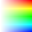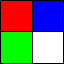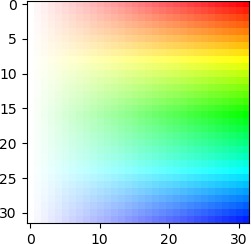14th October 2019

# Writing a (simple) PNG decoder might be easier than you think

The PNG Spec might seem daunting at first, but it's actually surprisingly easy to write a simple PNG decoder as long as we place some restrictions on our input PNG files, and we have a zlib decoder at hand.

For this exercise we shall use Python 3, which has zlib bindings available in its standard library that will make the job much easier for us.

## Restrictions

To avoid burdening ourselves with implementation details, we shall place some restrictions on our input PNG images:

• We only support 8 bit truecolor with alpha. In other words, a typical RGBA image where each pixel is a R,G,B,A tuple and each sample is one byte.
• We do not support interlacing.

As we can see, these restrictions aren't that bad and you should be able to decode some of your favorite images using this simple decoder.

## Starting off

For this exercise, we shall try to decode this RGBA PNG Image from PngSuite (LICENSE):Save this as `basn6a08.png` in your working directory.

To start off, we'll import the libraries we need, and open the PNG file for reading:

``````import struct
import zlib

f = open('basn6a08.png', 'rb')``````

We will use `struct` for parsing the PNG file, and `zlib` for decompressing image data later.

The first 8 bytes of a valid PNG file is the PNG signature, which is the byte string `b'\x89PNG\r\n\x1a\n'`. We start off by reading the first 8 bytes of the file and checking that it matches:

``````PngSignature = b'\x89PNG\r\n\x1a\n'
raise Exception('Invalid PNG Signature')``````

After the PNG signature comes a series of chunks, until the end of the file. Each chunk has the following fields:

 Length 4-byte unsigned integer giving the number of bytes in the chunk's data field. The length counts only the data field, not itself, the chunk type, or the CRC. Zero is a valid length. Chunk Type A sequence of 4 bytes defining the chunk type. Chunk Data The data bytes appropriate to the chunk type, if any. This field can be of zero length. CRC A 4-byte CRC (Cyclic Redundancy Code) calculated on the preceding bytes in the chunk, including the chunk type field and the chunk data fields, but not including the length field. The CRC can be used to check for corruption of the data.

Note that all integer fields in the PNG file format are stored in big endian order, that is, most significant byte first.

As such, we shall define a reusable function for reading chunks:

``````def read_chunk(f):
# Returns (chunk_type, chunk_data)
checksum = zlib.crc32(chunk_data, zlib.crc32(struct.pack('>4s', chunk_type)))
if chunk_crc != checksum:
raise Exception('chunk checksum failed {} != {}'.format(chunk_crc,
checksum))
return chunk_type, chunk_data``````

Now, let's read all the chunks of the file into a `chunks` list. The PNG spec requires the last chunk of the file to a chunk with chunk type `IEND`, so we can use it to determine when to break out of the loop:

``````chunks = []
while True:
chunks.append((chunk_type, chunk_data))
if chunk_type == b'IEND':
break``````

At this point, let's sanity-check the code we have written by printing the chunk types of the chunks we have read:

``print([chunk_type for chunk_type, chunk_data in chunks])``

The following line should be printed:

``[b'IHDR', b'gAMA', b'IDAT', b'IEND']``

As we can see, the PNG file contains 4 chunks:

• Firstly, the `IHDR` chunk, which contains important metadata such as the width/height of the image, bit depth etc. The PNG spec requires all PNG files to have `IHDR` as the first chunk.
• Next comes the `gAMA` chunk, which specifies gamma information. This is an optional chunk type, meaning that not all PNG files will specify it. For simplicity, we will simply ignore it for this exercise.
• The third chunk is the `IDAT` chunk, which contains image pixel data. The PNG spec requires all PNG files to have it.
• Finally, the last chunk is the `IEND` chunk, which signals the end of the file.

Generally speaking, the PNG spec defines two kinds of chunks: critical chunks --- chunks that are required to be present in the PNG file depending on certain conditions, and must be processed by the decoder, and ancillary chunks --- chunks that are optional and may be ignored by the decoder. You can easily tell what chunk types are critical and what chunk types are ancillary by looking at their first letter --- if it is upper-case (like `IDAT` and `IHDR`), it is critical, and if it is lower-case (like `gAMA`), it is ancillary. The PNG spec specifies a list of chunk types and how to interpret them. The list is not exhaustive, however, as we can also extend the PNG format with our own extension chunk types. This is how applications such as Adobe Fireworks are able to store all of their data inside regular png files, while still allowing them to be viewed with regular image viewers.

Now that we have all our chunks in the `chunks` list, let's process them.

## Processing the `IHDR` chunk

The `IHDR` chunk data is always 13 bytes, and contains the following fields:

Field nameField sizeDescription
Width4 bytes4-byte unsigned integer. Gives the image dimensions in pixels. Zero is an invalid value.
Height4 bytes
Bit depth1 byteA single-byte integer giving the number of bits per sample or per palette index (not per pixel). Only certain values are valid (see below).
Color type1 byteA single-byte integer that defines the PNG image type. Valid values are 0 (grayscale), 2 (truecolor), 3 (indexed-color), 4 (greyscale with alpha) and 6 (truecolor with alpha).
Compression method1 byteA single-byte integer that indicates the method used to compress the image data. Only compression method 0 (deflate/inflate compression with a sliding window of at most 32768 bytes) is defined in the spec.
Filter method1 byteA single-byte integer that indicates the preprocessing method applied to the image data before compression. Only filter method 0 (adaptive filtering with five basic filter types) is defined in the spec.
Interlace method1 byteA single-byte integer that indicates whether there is interlacing. Two values are defined in the spec: 0 (no interlace) or 1 (Adam7 interlace).

The valid bit depths and color types are defined as follows:

PNG Image TypeColor typeAllowed bit depthsInterpretation
Grayscale01, 2, 4, 8, 16Each pixel is a grayscale sample.
Truecolor28, 16Each pixel is a R,G,B triple.
Indexed-color31,2,4,8Each pixel is a palette index; a `PLTE` chunk shall appear.
Grayscale with alpha48, 16Each pixel is a grayscale sample followed by an alpha sample.
Truecolor with alpha68, 16Each pixel is a R,G,B triple followed by an alpha sample.

To start off, we'll grab the `IHDR` chunk and parse its fields:

``````_, IHDR_data = chunks # IHDR is always first chunk
width, height, bitd, colort, compm, filterm, interlacem = struct.unpack('>IIBBBBB', IHDR_data)``````

Next, we'll perform some sanity checking. As mentioned in the tables above, the PNG spec only supports `0` as the compression method and `0` as the filter method.

``````if compm != 0:
raise Exception('invalid compression method')
if filterm != 0:
raise Exception('invalid filter method')``````

Next, we'll apply some of our own restrictions. As mentioned above we only accept images with Truecolor with alpha (color type = `6`), a bit depth of 8 , and no interlacing (interlace method = `0`):

``````if colort != 6:
raise Exception('we only support truecolor with alpha')
if bitd != 8:
raise Exception('we only support a bit depth of 8')
if interlacem != 0:
raise Exception('we only support no interlacing')``````

Finally, let's sanity-check our code by printing the width and height of the image:

``print(width, height)``

The following should be printed out:

``32 32``

which matches the dimensions of the image.

Now we have processed the image header, and can now move on to processing the image data.

## Processing `IDAT` chunk(s)

At this point, we are ready to decode the image data to get back the raw pixel data.

To start, let's first discuss how raw pixel data is transformed into the data that is stored in the PNG file.

An image consists of scanlines, which are individual rows of pixels. Since we are dealing with a RGBA 8-bit image, each pixel consists of RGBA samples (in the order of R, G, B, then A), where each sample is 1 unsigned byte.

For example, let's say we have this 2x2 image:• The first scanline consists of 2 pixels with RGBA tuples and .
• The second scanline consists of 2 pixels with RGBA tuples and

As such, when represented using Python lists, the raw image data looks something like this:

``````raw_image_data = [
[255, 0, 0, 255, 0, 0, 255, 255], # First scanline
[0, 255, 0, 255, 255, 255, 255, 255], # Second scanline
]``````

Next, the encoder will perform filtering on each scanline. Filtering is an operation specified by the PNG spec that is applied on the raw image pixel data in order to allow the pixel data to be compressed more effectively. We'll cover filtering in more detail later on, but what's important to note is that there are different filter types, and each scanline will be prefixed with a byte indicating which filter type was used to filter the scanline.

For example, filter type `0` is the identity filter --- the result of filtering a scanline is the original scanline itself. If we filter the two scanlines of the original raw_image_data, the resulting filtered_image_data looks something like this:

``````filtered_image_data = [
[0, 255, 0, 0, 255, 0, 0, 255, 255], # First scanline, first item (0) specifies filter type 0
[0, 0, 255, 0, 255, 255, 255, 255, 255], # Second scanline, first item (0) specifies filter type 0
]``````

Finally, the scanlines are concatenated together into one contiguous bytearray, compressed in accordance with the zlib spec, and then packaged into `IDAT` chunks.

As such, to decode the image, we'll just need to do the above operations in reverse!

### Consolidating `IDAT` data

The image pixel data is stored in `IDAT` chunks. The PNG spec allows for the image data to be split across multiple `IDAT` chunks, so we'll need to concatenate them together as follows:

``IDAT_data = b''.join(chunk_data for chunk_type, chunk_data in chunks if chunk_type == b'IDAT')``

Tip

The PNG spec requires `IDAT` chunks to appear consecutively in the file with no other intervening chunks. We don't take advantage of this constraint here, but it might be useful when you are writing your own PNG decoder.

### Decompressing `IDAT` data

The `IDAT` chunk data is compressed, so we'll need to decompress it with the zlib library as follows:

``IDAT_data = zlib.decompress(IDAT_data)``

### Reconstructing pixel data

At this point, we have the filtered pixel data! As mentioned above, it consists of individual scanlines of filtered pixel data. Each scanline of filtered pixel data is prefixed with a byte indicating the filter type which was used to filter the scanline.

Let's first double-check that the shape of our data is correct by examining its size:

``print(len(IDAT_data))``

This prints:

``4128``

As calculated above, the width of our image is 32 and the height of our image is 32. It is a RGBA 8-bit image, which means each pixel is 4 bytes long. Furthermore, each scanline is prefixed with 1 filter type byte. So, calculating the expected size of `IDAT_data`:

So, everything seems correct and we are good to go do our reverse filtering!

Filters operate on the byte sequence formed by the scanline. The exhaustive list of filter types is:

TypeNameFilter FunctionReconstruction Function
0None`Filt(x) = Orig(x)``Recon(x) = Filt(x)`
1Sub`Filt(x) = Orig(x) - Orig(a)``Recon(x) = Filt(x) + Recon(a)`
2Up`Filt(x) = Orig(x) - Orig(b)``Recon(x) = Filt(x) + Recon(b)`
3Average`Filt(x) = Orig(x) - floor((Orig(a) + Orig(b)) / 2)``Recon(x) = Filt(x) + floor((Recon(a) + Recon(b)) / 2)`
4Paeth`Filt(x) = Orig(x) - PaethPredictor(Orig(a), Orig(b), Orig(c))``Recon(x) = Filt(x) + PaethPredictor(Recon(a), Recon(b), Recon(c))`

where:

• `x` is the byte being filtered
• `a` is the byte corresponding to `x` in the pixel immediately before the pixel containing `x` (or 0 if such a pixel is out of bounds of the image)
• `b` is the byte corresponding to `x` in the previous scanline (or 0 if such a scanline is out of bounds of the image)
• `c` is the byte corresponding to `b` in the pixel immediately before the pixel containing `b` (or 0 if such a pixel is out of bounds of the image)

and `PaethPredictor` is defined as an algorithm as follows:

``````def PaethPredictor(a, b, c):
p = a + b - c
pa = abs(p - a)
pb = abs(p - b)
pc = abs(p - c)
if pa <= pb and pa <= pc:
Pr = a
elif pb <= pc:
Pr = b
else:
Pr = c
return Pr``````

Since we are re-constructing the raw image pixels from the filtered image pixels, we only need to consider the reconstruction functions. The PNG spec specifies that unsigned arithmetic modulo 256 is used (with the exception of the Average filter which should be done without overflow), so that both the inputs and outputs fit into bytes. Since `a mod 256 +/- b mod 256 = (a +/- b) mod 256`, we'll simply do a one-time truncation at the end.

First, let's create a list `Recon` to hold our reconstructed pixel data:

``Recon = []``

Next, we need a way to calculate `Recon(a)`, `Recon(b)` and `Recon(c)` as they are needed to implement the reconstruction functions. Calculating them is simple once we define the `bytesPerPixel` and `stride` of the image, which is:

``````bytesPerPixel = 4
stride = width * bytesPerPixel``````

Then, we let `r` be the scanline index of the byte being reconstructed and `c` be the index of that byte along the scanline, we can implement `Recon(a)`, `Recon(b)` and `Recon(c)` as:

``````def Recon_a(r, c):
return Recon[r * stride + c - bytesPerPixel] if c >= bytesPerPixel else 0

def Recon_b(r, c):
return Recon[(r-1) * stride + c] if r > 0 else 0

def Recon_c(r, c):
return Recon[(r-1) * stride + c - bytesPerPixel] if r > 0 and c >= bytesPerPixel else 0``````

And now we can finally write the loop which reconstructs the pixel data from `IDAT_data` and puts that into `Recon`:

``````i = 0
for r in range(height): # for each scanline
filter_type = IDAT_data[i] # first byte of scanline is filter type
i += 1
for c in range(stride): # for each byte in scanline
Filt_x = IDAT_data[i]
i += 1
if filter_type == 0: # None
Recon_x = Filt_x
elif filter_type == 1: # Sub
Recon_x = Filt_x + Recon_a(r, c)
elif filter_type == 2: # Up
Recon_x = Filt_x + Recon_b(r, c)
elif filter_type == 3: # Average
Recon_x = Filt_x + (Recon_a(r, c) + Recon_b(r, c)) // 2
elif filter_type == 4: # Paeth
Recon_x = Filt_x + PaethPredictor(Recon_a(r, c), Recon_b(r, c), Recon_c(r, c))
else:
raise Exception('unknown filter type: ' + str(filter_type))
Recon.append(Recon_x & 0xff) # truncation to byte``````

And we have finished decoding the image! To check its correctness, let's plot it using matplotlib:

``````import matplotlib.pyplot as plt
import numpy as np
plt.imshow(np.array(Recon).reshape((height, width, 4)))
plt.show()``````

Result:which looks correct!

## Full source code

``````import zlib
import struct

f = open('basn6a08.png', 'rb')

PngSignature = b'\x89PNG\r\n\x1a\n'
raise Exception('Invalid PNG Signature')

# Returns (chunk_type, chunk_data)
chunk_actual_crc = zlib.crc32(chunk_data, zlib.crc32(struct.pack('>4s', chunk_type)))
if chunk_expected_crc != chunk_actual_crc:
raise Exception('chunk checksum failed')
return chunk_type, chunk_data

chunks = []
while True:
chunks.append((chunk_type, chunk_data))
if chunk_type == b'IEND':
break

_, IHDR_data = chunks # IHDR is always first chunk
width, height, bitd, colort, compm, filterm, interlacem = struct.unpack('>IIBBBBB', IHDR_data)
if compm != 0:
raise Exception('invalid compression method')
if filterm != 0:
raise Exception('invalid filter method')
if colort != 6:
raise Exception('we only support truecolor with alpha')
if bitd != 8:
raise Exception('we only support a bit depth of 8')
if interlacem != 0:
raise Exception('we only support no interlacing')

IDAT_data = b''.join(chunk_data for chunk_type, chunk_data in chunks if chunk_type == b'IDAT')
IDAT_data = zlib.decompress(IDAT_data)

def PaethPredictor(a, b, c):
p = a + b - c
pa = abs(p - a)
pb = abs(p - b)
pc = abs(p - c)
if pa <= pb and pa <= pc:
Pr = a
elif pb <= pc:
Pr = b
else:
Pr = c
return Pr

Recon = []
bytesPerPixel = 4
stride = width * bytesPerPixel

def Recon_a(r, c):
return Recon[r * stride + c - bytesPerPixel] if c >= bytesPerPixel else 0

def Recon_b(r, c):
return Recon[(r-1) * stride + c] if r > 0 else 0

def Recon_c(r, c):
return Recon[(r-1) * stride + c - bytesPerPixel] if r > 0 and c >= bytesPerPixel else 0

i = 0
for r in range(height): # for each scanline
filter_type = IDAT_data[i] # first byte of scanline is filter type
i += 1
for c in range(stride): # for each byte in scanline
Filt_x = IDAT_data[i]
i += 1
if filter_type == 0: # None
Recon_x = Filt_x
elif filter_type == 1: # Sub
Recon_x = Filt_x + Recon_a(r, c)
elif filter_type == 2: # Up
Recon_x = Filt_x + Recon_b(r, c)
elif filter_type == 3: # Average
Recon_x = Filt_x + (Recon_a(r, c) + Recon_b(r, c)) // 2
elif filter_type == 4: # Paeth
Recon_x = Filt_x + PaethPredictor(Recon_a(r, c), Recon_b(r, c), Recon_c(r, c))
else:
raise Exception('unknown filter type: ' + str(filter_type))
Recon.append(Recon_x & 0xff) # truncation to byte

import matplotlib.pyplot as plt
import numpy as np
plt.imshow(np.array(Recon).reshape((height, width, 4)))
plt.show()``````